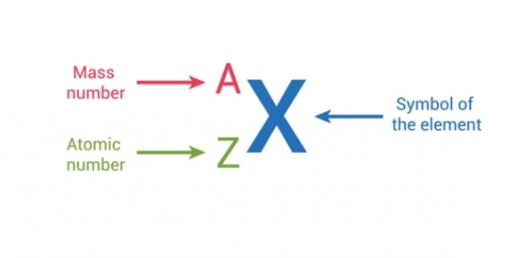# Inside Of Atom - Atomic Number & Mass Quiz #2

10 Questions | Attempts: 398
ShareSettingsDo you know anything about the number of atoms and packing efficiency? Packing fraction is the fraction of size in a crystal structure that is inhabited by constituent particles. The atomic packing factor of a unit cell is relevant to the study of items, explaining many properties of materials. If you would like to understand more about this topic, try this quiz.

• 1.
If lithium has an atomic number of 3 and a mass number of 7, the correct number of protons, neutrons, and electrons, respectively, is:
• A.

3,4,3

• B.

4,3,3

• C.

3,3,4

• D.

4,4,3

• 2.
If Magnesium has an atomic number of 12 and a mass number of 24, the correct number of protons, neutrons, and electrons, respectively, is:
• A.

8,10,12

• B.

12,12,12

• C.

12,24,12

• D.

24,12,12

• 3.
If Zinc has an atomic number of 30 and a mass number of 65, the correct number of protons, neutrons, and electrons, respectively, is:
• A.

30,35,35

• B.

30,35,30

• C.

35,30,35

• D.

95,65,30

• 4.
If Bromine has an atomic number of 35 and has 45 neutrons, what is its mass number?
• A.

35

• B.

45

• C.

10

• D.

80

• 5.
If Aluminum has an atomic number of 13 and has 14 neutrons, what is its mass number?
• A.

1

• B.

27

• C.

13

• D.

14

• 6.
If Uranium's atomic number is 92 and it has 10 neutrons, how many protons does it have?
• A.

102

• B.

92

• C.

10

• D.

82

• 7.
If Sodium has an atomic number of 11 and a mass number of 23, how many protons, neutrons, and electrons does it have, respectively?
• A.

11,12,12

• B.

12,11,12

• C.

11,12,11

• D.

12,12,11

• 8.
If Phosphorus has an atomic mass of 30 and 15 neutrons, how many protons does it has?
• A.

15

• B.

45

• C.

30

• D.

60

• 9.
If carbon has an atomic mass of 12 and 6 neutrons, how many protons and electrons does it have, respectively?
• A.

6,6

• B.

6,12

• C.

12,6

• D.

24,6

• 10.
If Potassium has 19 protons, 19 electrons, and 20 neutrons, what is its atomic mass?
• A.

1

• B.

58

• C.

39

• D.

29

## Related TopicsBack to top
×

Wait!
Here's an interesting quiz for you.